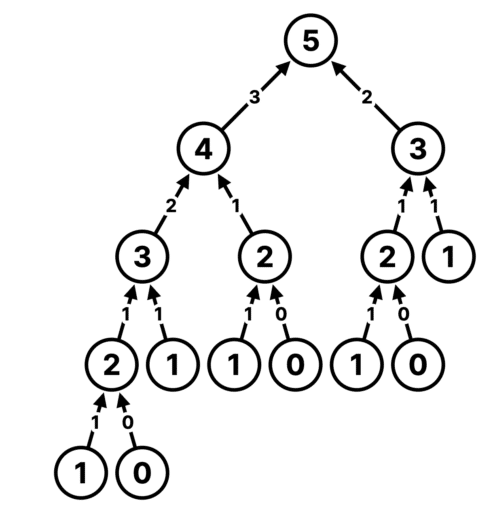In our previous article, we discussed the thread pool’s sizing. We stipulated that if work is computational, we don’t need more threads than the available number of processors. We will discuss this idea a bit further in this article.

We all know how to compute the Fibonacci series. The simple code is given below-

```public static long fib(int n) {
if (n < 2) {
return n;
} else {
return fib(n - 2) + fib(n - 2);
}
}```

Over here, we have used recursion to solve the Fibonacci series. To find the Fibonacci number of n, we must know the previous two. Each of them again will require to calculate the previous two. The process will continue until it reaches to the bases case and then we have a result.

The above code is single-threaded and sequential, which is fine for our usual use cases. But all the modern computer has more than 1 processor. If our program uses only one, that is certainly a waste of valuable resources. If we could turn this into a multithreaded program, we could use all the processorss available in the machine.

Let’s do that.

```package ca.bazlur;

import java.util.concurrent.ExecutionException;
import java.util.concurrent.ExecutorService;
import java.util.concurrent.Executors;
import java.util.concurrent.Future;

public class Playground6 {

public static void main(String[] args) throws ExecutionException, InterruptedException {

System.out.println("fifthFibonacciNumber = " + fifthFibonacciNumber.get());

}

public static long fib(int n, ExecutorService threadPool)
throws ExecutionException, InterruptedException {
if (n < 2) {
return n;
} else {
return prev.get() + prevPrev.get();
}
}
}```

Note that this code is just an experiment. Please don’t use this in a production environment.

Let me explain the code a bit.

First, we created a thread pool with the number of available processors in my machine.

To find how many processors are available on a computer, we can use the following method –

`Runtime.getRuntime().availableProcessors()`

Then we created a Callable with a lambda expression and passed it to the thread pool. The lambda expression calls the fib() method.

As we know, fibonacci series is – 0, 1,1, 2,3,5,8….

Which is mathmatically- `f(n) = f(n-1) + f(n-2)`

We usually use recursion for that. We know recursion must have a base case; over here, it is –  if the n is less than 2, then we return n.

If n is greater than 2, we must calculate from the last two Fibonacci numbers.

We can calculate the last two Fibonacci numbers in two tasks with Callable and submit them back to the ThreadPool.

That’s what we did.

The caveat is that this method won’t return any value until the two last Fibonacci numbers are calculated unless it reaches to the base case. So if this fib() runs on a worker thread in a pool, it must wait until it gets the two results.

And here comes the problem.

If we want to calculate a large number, like 10 in the above program, the program will yield no result. It will create a deadlock like situation (this is not a deadlock, but it appears so)

While you experiment it, let me explain what happens to it.

Let’s assume we have 4 processors, and we want create a ThreadPool with four threads. And we want to calculate Fibonacci of 5.

With the above code, how many tasks will be created?

The recursion tree will look like this.We count that we will have 15 tasks. The top task, which is 5, will create two new tasks and wait for them to finish.

Since we have four threads, one will go into a waiting state. We now have only three left. Task 4 and 5 will be picked two of these threads. But each of them will create two more tasks each wait for them to finish. So now 3 threads are in a waiting state. Only one is available.

We could have solved this problem if we had more thread in the pool.

Interestingly if we use CachedThreadPool, the problem goes away,

``var threadPool = Executors.newCachedThreadPool();``

Because CachedThreadPool creates a thread on the fly whenever it requires one. The only caveat is that we cannot create unlimited threads. If we want to calculate the Fibonacci number of a large number, we will have more tasks, and more threads will be waiting.

So it’s a genuine problem. And we concluded that a thread while performing a task in the executors cannot create a child task and wait for the child task to finish.

However, when there is a problem, there is a solution. Precisely for this sort of problem, we have  a unique factory method in Executors—

`var threadPool = Executors.newWorkStealingPool();`

This method returns an instance of ForkJoinPool. This pool allows its threads to create new tasks and suspend their current tasks when they wait for their child tasks to finish.

If you replace the above code with this ForkJoinPool, we will not have any problem running it. There is a special algorithm called WorkStealing in The ForkJoinPool.

Why not you try it?

While I give you a glimpse of ForkJoinPool, we will discuss it in more detail in the following article.

Until then, cheers!

##### A N M Bazlur Rahman
Website | + posts

Sr Software Engineer @contrastsec, Founder/JUG Leader @jugbdorg

Books: http://bit.ly/3JvrsA0 http://amzn.to/3zByoHa
#Java #jvm #develoepr, #author

This site uses Akismet to reduce spam. Learn how your comment data is processed.